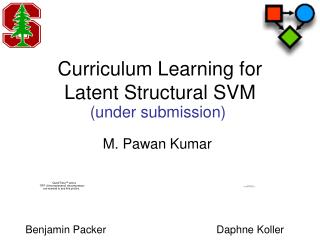DownloadDownload PresentationCurriculum Learning for Latent Structural SVM

# Curriculum Learning for Latent Structural SVM

Download Presentation## Curriculum Learning for Latent Structural SVM

- - - - - - - - - - - - - - - - - - - - - - - - - - - E N D - - - - - - - - - - - - - - - - - - - - - - - - - - -
##### Presentation Transcript

1. Curriculum Learning forLatent Structural SVM (under submission) M. Pawan Kumar Benjamin Packer Daphne Koller

2. Aim To learn accurate parameters for latent structural SVM Input x Output y Y Hidden Variable h  H “Deer” Y = {“Bison”, “Deer”, ”Elephant”, “Giraffe”, “Llama”, “Rhino” }

3. Aim To learn accurate parameters for latent structural SVM Feature (x,y,h) (HOG, BoW) Parameters w (y*,h*) = maxyY,hH wT(x,y,h)

4. Motivation Math is for losers !! Real Numbers Imaginary Numbers eiπ+1 = 0 FAILURE … BAD LOCAL MINIMUM

5. Motivation Euler was a Genius!! Real Numbers Imaginary Numbers eiπ+1 = 0 SUCCESS … GOOD LOCAL MINIMUM Curriculum Learning: Bengio et al, ICML 2009

6. Motivation Start with “easy” examples, then consider “hard” ones Simultaneously estimate easiness and parameters Easiness is property of data sets, not single instances Easy vs. Hard Expensive Easy for human  Easy for machine

7. Outline • Latent Structural SVM • Concave-Convex Procedure • Curriculum Learning • Experiments

8. Latent Structural SVM Felzenszwalb et al, 2008, Yu and Joachims, 2009 Training samples xi Ground-truth label yi Loss Function (yi, yi(w), hi(w))

9. Latent Structural SVM (yi(w),hi(w)) = maxyY,hH wT(x,y,h) min ||w||2 + C∑i(yi, yi(w), hi(w)) Non-convex Objective Minimize an upper bound

10. Latent Structural SVM (yi(w),hi(w)) = maxyY,hH wT(x,y,h) min ||w||2 + C∑i i maxhiwT(xi,yi,hi) - wT(xi,y,h) ≥ (yi, y, h) - i Still non-convex Difference of convex CCCP Algorithm - converges to a local minimum

11. Outline • Latent Structural SVM • Concave-Convex Procedure • Curriculum Learning • Experiments

12. Concave-Convex Procedure Start with an initial estimate w0 hi = maxhH wtT(xi,yi,h) Update Update wt+1 by solving a convex problem min ||w||2 + C∑i i wT(xi,yi,hi) - wT(xi,y,h) ≥ (yi, y, h) - i

13. Concave-Convex Procedure Looks at all samples simultaneously “Hard” samples will cause confusion Start with “easy” samples, then consider “hard” ones

14. Outline • Latent Structural SVM • Concave-Convex Procedure • Curriculum Learning • Experiments

15. Curriculum Learning REMINDER Simultaneously estimate easiness and parameters Easiness is property of data sets, not single instances

16. Curriculum Learning Start with an initial estimate w0 hi = maxhH wtT(xi,yi,h) Update Update wt+1 by solving a convex problem min ||w||2 + C∑i i wT(xi,yi,hi) - wT(xi,y,h) ≥ (yi, y, h) - i

17. Curriculum Learning min ||w||2 + C∑i i wT(xi,yi,hi) - wT(xi,y,h) ≥ (yi, y, h) - i

18. Curriculum Learning vi {0,1} min ||w||2 + C∑i vii wT(xi,yi,hi) - wT(xi,y,h) ≥ (yi, y, h) - i Trivial Solution

19. Curriculum Learning vi {0,1} min ||w||2 + C∑i vii - ∑ivi/K wT(xi,yi,hi) - wT(xi,y,h) ≥ (yi, y, h) - i Large K Medium K Small K

20. Curriculum Learning Biconvex Problem vi [0,1] min ||w||2 + C∑i vii - ∑ivi/K wT(xi,yi,hi) - wT(xi,y,h) ≥ (yi, y, h) - i Large K Medium K Small K

21. Curriculum Learning Start with an initial estimate w0 hi = maxhH wtT(xi,yi,h) Update Update wt+1 by solving a convex problem min ||w||2 + C∑i vii - ∑i vi/K wT(xi,yi,hi) - wT(xi,y,h) ≥ (yi, y, h) - i Decrease K  K/

22. Outline • Latent Structural SVM • Concave-Convex Procedure • Curriculum Learning • Experiments

23. Object Detection Input x - Image Output y Y Latent h - Box  - 0/1 Loss Y = {“Bison”, “Deer”, ”Elephant”, “Giraffe”, “Llama”, “Rhino” } Feature (x,y,h) - HOG

24. Object Detection Mammals Dataset 271 images, 6 classes 90/10 train/test split 5 folds

25. Object Detection Curriculum CCCP

26. Object Detection Curriculum CCCP

27. Object Detection Curriculum CCCP

28. Object Detection Curriculum CCCP

29. Object Detection Objective value Test error

30. Handwritten Digit Recognition Input x - Image Output y Y Latent h - Rotation  - 0/1 Loss MNIST Dataset Y = {0, 1, … , 9} Feature (x,y,h) - PCA + Projection

31. Handwritten Digit Recognition C C C - Significant Difference

32. Handwritten Digit Recognition C C C - Significant Difference

33. Handwritten Digit Recognition C C C - Significant Difference

34. Handwritten Digit Recognition C C C - Significant Difference

35. Motif Finding Input x - DNA Sequence Output y Y Y = {0, 1} Latent h - Motif Location  - 0/1 Loss Feature (x,y,h) - Ng and Cardie, ACL 2002

36. Motif Finding UniProbe Dataset 40,000 sequences 50/50 train/test split 5 folds

37. Average Hamming Distance of Inferred Motifs Motif Finding

38. Motif Finding Objective Value

39. Motif Finding Test Error

40. Noun Phrase Coreference Input x - Nouns Output y - Clustering Latent h - Spanning Forest over Nouns Feature (x,y,h) - Yu and Joachims, ICML 2009

41. Noun Phrase Coreference MUC6 Dataset 60 documents 1 predefined fold 50/50 train/test split

42. Noun Phrase Coreference MITRE Loss Pairwise Loss - Significant Improvement - Significant Decrement

43. Noun Phrase Coreference MITRE Loss Pairwise Loss

44. Noun Phrase Coreference MITRE Loss Pairwise Loss

45. Summary • Automatic Curriculum Learning • Concave-Biconvex Procedure • Generalization to other Latent models • Expectation-Maximization • E-step remains the same • M-step includes indicator variables vi